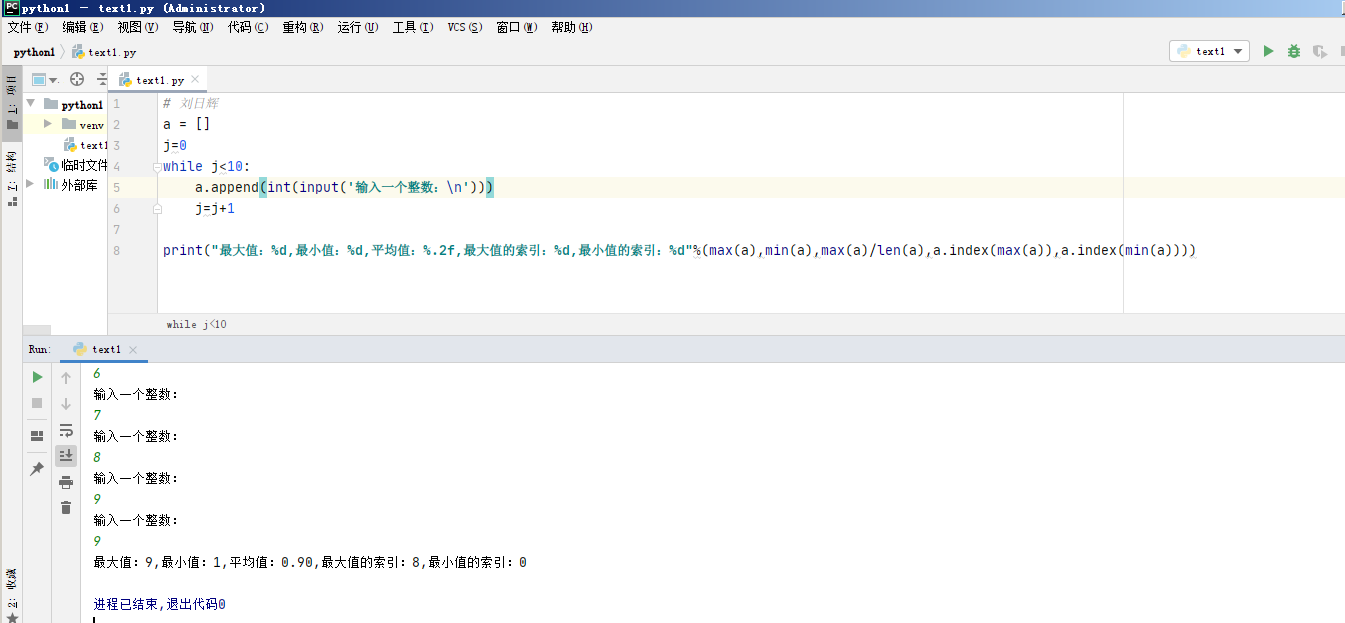• 微信公众号：美女很有趣。 工作之余，放松一下，关注即送10G+美女照片！

2周前 (04-06) 5次浏览

# 一、题目

1、从外界获取10个值，输出最大值、最小值、平均值；
2、从外界获取10个值，输出最大值的索引、最小值的索引

# 二、解答

## 2.1从外界获取数据

input()函数

a=[]

a.append(int(input()))

a=[1,2,3,4,5,6,7,8,9,10]

a=int(input())

i=0;

while i<10:

a.append(int(input())) #如果列表一开始是空的

a[i]=int(input())#如果列表一开始就有了数据

i=i+1

theMax=a

if a>theMax:

theMax=a

if a>theMax:

theMax=a

…以此到：

if(a>theMax):

theMax=a

j=0;

while j<len(a):

if j>theMax:

theMax=j

j++

theMin=a

if a<theMin:

theMin=a

if a<theMin:

theMin=a

…以此到：

if(a<theMin):

theMin=a

j=0;

while j<len(a):

if j<theMin:

theMin=j

j++

## 2.3求平均值

sum=a+a+a+a+a+a+a+a+a+a

sum=0

for z in a:

sum=sum+z

theAvg=sum/len(a)

## 2.4求最值索引

indexMax=0

indexMin=0

``````indexMax=0
indexMin=0
for j in a:
if j>theMax:#如果发现有一个值比theMax还大
theMax=j
indexMax=a.index(j)#通过函数获取索引
if theMin>j:#如果发现有一个值比theMin还小
theMin=j
indexMin=a.index(j)#通过函数获取索引
theSum=theSum+j``````

``````q=0#记录索引
for j in a:
if j>theMax:#如果发现有一个值比theMax还大
theMax=j
indexMax=q
if theMin>j:#如果发现有一个值比theMin还小
theMin=j
indexMin = q
theSum=theSum+j
q = q + 1``````

``````indexMax=0
indexMin=0
i=0#记录索引
while i<len(a):
if a[i]>theMax:
theMax=a[i]
indexMax=i
if theMin>a[i]:
theMin=a[i]
indexMin=i
theSum=theSum+a[i]
i=i+1``````

# 三、代码© 著作权归作者所有

0

0 收藏

QQ

### 作者的其它热门文章

《C语言程序设计》期末考点1：编写目录提示墙